# Maths - Fibre/Fiber

### Fibre

Or Fiber (alternative spelling).

Fibration captures the idea of one category indexed over another category. It does this in a categorical way, that is, defined in terms of arrows.

A fibre is a more general (weaker form) of:

• a projection
• a pullback or pushout (fiber (co)product) - indexed and indexing category are the same.
• a comma category.
• one quantity defined 'over' another such as a field space.
• a base space.
• sorting or partitioning.
 If we take a mapping from A to B (in this case surjective) We might think of this as partitioning or indexing A into disjoint subsets but there is no unique arrow going the other direction (from B to A).We can put the elements into subsets, that is, if A was a set then change it to a set of sets. I have changed the name of 'B' to 'I' because we want to think of it as an indexing set. We can now think of 'A' as a disjoint set of dots. In general the mapping need not be surjective and some sets in A may be empty.Since we have put the elements into subsets we can reverse the arrows.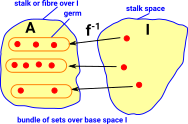We can define the sets in A in terms of the inverse mapping.

### Category of bundles over 'I'

We can turn this around and start with 'I', We can then construct a whole category of bundles that could map to it.

Construct in Bn(I) Corresponding construct in set
Bn(I)=
SetI
category of bundles over I the comma category of functions with codomain I
pullback object (P,j) bundle of pullbacks from set
subobject classifier bundle of two element sets
Sections
Products
Exponentials

In Bn(I) objects are pairs, consisting of an object and a map from that object to I. Represented as: (A,f) where f:A->I

 If we have multiple bundles over I then, we can define morphisms in the category, so that this diagram commutes.In terms of its internal objects:
 Here we add another stalk 'B' and add a morphism: A->B. In order for the diagram to commute then the internal arrows can only go from an element in a germ in 'A' to an element in the corresponding germ in 'B'.We could re-draw this as a set of fibres, within a bigger fibre, indexed by I. The inner fibre can be permutated and the outer fibre can be permutated but the inner strands can't be moved between outer fibres.So if we take the example of the Möbius band, that we looked at on the page here, we can see it as a fibre. It is arbitrary where it starts and ends so it is only where we take the local view that we see it as two fibres.### Preservation of Product

 A projection along a fibre looks, locally but not necessarily globally, like a product space. The diagram on the right is intended to show a non-linear space but locally it is still a product (The contours cross at right angles). This can be represented by a pullback square, as discussed on the page here.### Fibre Bundle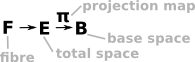π is a 'continuous surjective map' that behaves locally like B×F->B.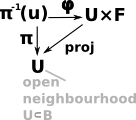### Terminology

The terminology is somewhat 'Botanical' suggesting fibres growing out of I:

• The set Ai is called the stalk or fibre over i.
• The members of Ai are called the germs at i.
• The whole structure is called a bundle of sets over the base space I.
• The set A is called the stalk space of the bundle.

In geometric terms: I is a base space and A is a projection.

### Fibrations of Graphs

theory: topological graph theory

 undirected graph: covering projection directed graph: fibration (weaker form of covering projection)
 Here is an example for directed multigraphs. Each node in the top graph maps to the bottom graph (fibration). The corresponding node always has the same number and colour of incoming arcs (but not necessarily outgoing arcs). This gives some sort of local invariance.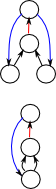We can make a mapping between these graphs.We can't reverse the mapping completely because we can't have one-to-many in a function but we can map to sets. This gives a fibre bundle.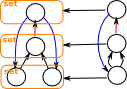### Types

Here we look at applications of fibre bundles to type theory (discussed on page here).

 Here we look at a type, not like a set as a membership relationship, but more like a projection. So if we know that something is 1 or 2 then that tells us something about it, if we know that something is a number then that still gives us information about it but less than if we knew its value.#### Dependent Types

 The situation where a type depends on the value of another type (called a dependent type) is modeled by fibre bundles. So a model of a vector category depends on its dimension:### Fibre Concept In Topology

In topology the concept of 'nearness' can be defined in a looser way than metric spaces, this is done by using 'open sets' as described here.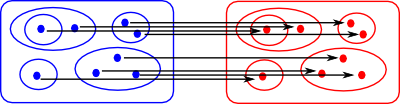A fiber is such a topological space which is parameterized by another (called a base).

• Fiber bundle - map between fibres in the same space.
• Fibration - fibers need not be the same space

Fibrations do not necessarily have the local Cartesian product structure that defines the more restricted fiber bundle case, but something weaker that still allows 'sideways' movement from fiber to fiber.

### Sheaf

A sheaf is a tool for systematically tracking locally defined data attached to the open sets of a topological space.

 Sheaves, with mappings which preserve this open set structure, form a category. This open set structure can be extended to groups, abelian groups, or commutative rings. To make it more general and apply it to more general categories we use the concept of presheaf which uses 'restriction morphisms'. More about presheaf on page here.### Enriched Category

No unique functor between objects but multiple functors (hom-set).

Allows us to represent a metric space as a category.

### Fibrations

 Relationship between fibrationsWhere:

• Eff = effective topos

### As a Pullback

See pullback page.

Wikipedia: Pullback bundle

Where I can, I have put links to Amazon for books that are relevant to the subject, click on the appropriate country flag to get more details of the book or to buy it from them.The Princeton Companion to Mathematics - This is a big book that attempts to give a wide overview of the whole of mathematics, inevitably there are many things missing, but it gives a good insight into the history, concepts, branches, theorems and wider perspective of mathematics. It is well written and, if you are interested in maths, this is the type of book where you can open a page at random and find something interesting to read. To some extent it can be used as a reference book, although it doesn't have tables of formula for trig functions and so on, but where it is most useful is when you want to read about various topics to find out which topics are interesting and relevant to you.